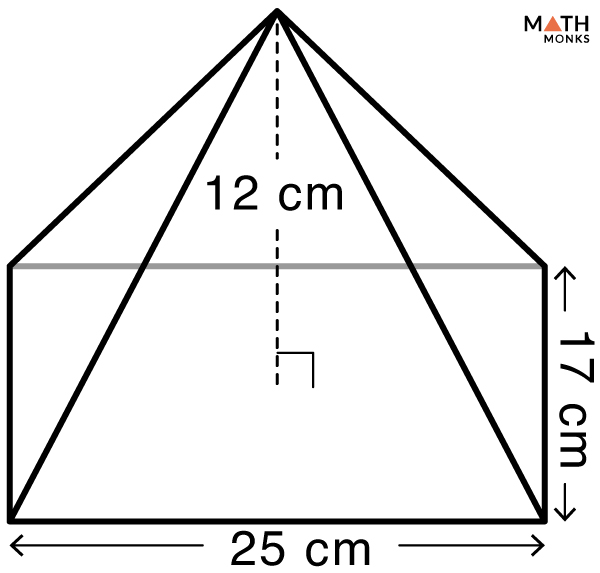# Volume of a Rectangular Pyramid

The volume of a rectangular pyramid is the space it occupies in a 3-dimensional plane. It is the capacity of a rectangular pyramid or the number of unit cubes that can be fit into it. The volume is expressed in cubic units such as m3, cm3, mm3, and in3.

## Formula

The formula to calculate the volume of an oblique or a right rectangular pyramid is the same as we consider the perpendicular height of the pyramid for both cases. The general formula to calculate the volume of a rectangular pyramid is:

Volume (V) = ${\dfrac{1}{3}Bh}$, here B = base area, h = height

The specific formula is given below:

Let us solve some examples to understand the above concept better.

## Solved Examples

Find the volume of a rectangular pyramid with a base length of 15 cm, a base width of 9 cm, and a height of 21 cm.

Solution:

As we know,
Volume (V) = ${\dfrac{1}{3}lwh}$, here l = 15 cm, w = 9 cm, h = 21 cm
V = ${\dfrac{1}{3}\times 15\times 9\times 21}$
= 945 cm3Find the volume of a right rectangular pyramid given in the figure.

Solution:

As we know,
Volume (V) = ${\dfrac{1}{3}lwh}$, here l = 25 cm, w = 17 cm, h = 12 cm
V = ${\dfrac{1}{3}\times 25\times 17\times 12}$
= 1700 cm3

Let us learn to calculate the volume of a truncated rectangular pyramid pond by solving an example.

Finding the volume of a truncated rectangular pyramid when BOTTOM BASE LENGTH, BOTTOM BASE WIDTH, TOP BASE LENGTH, TOP BASE WIDTH, and HEIGHT are knownFind the volume of a truncated rectangular pyramid pond given in the figure.

Solution:

Here, we will use the volume formula of truncated rectangular pyramid.
Volume (V) = ${\dfrac{Ab+aB+2\left( ab+AB\right) }{6}\times h}$, here A = 18 m, B = 16 m, a = 9 m, b = 8 m, h = 10 m
V = ${\dfrac{18\times 8+9\times 16+2\left( 9\times 8+18\times 16\right) }{6}\times 10}$
= 1680 m3